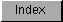```
The IEEE double-precision basic math library contains entries for the
basic IEEE mathematics functions, such as add, subtract, and divide. This
library resides on disk and is opened by calling OpenLibrary() with
"mathieeedoubbas.library" as the argument.  Do not share the library base
pointer between tasks -- see note at beginning of chapter for details.

#include <exec/types.h>
#include <libraries/mathieeedp.h>

#include <clib/mathdoubbas_protos.h>

struct Library *MathIeeeDoubBasBase;

VOID main()
{
/* do not share base pointer between tasks. */
if (MathIeeeDoubBasBase = OpenLibrary("mathieeedoubbas.library", 34))
{
. . .

CloseLibrary(MathIeeeDoubBasBase);
}
else printf("Can't open mathieeedoubbas.library\n");
}

The global variable MathIeeeDoubBasBase is used internally for all future
library references.

If an 680X0/68881/68882 processor combination is available, it will be
used by the IEEE basic library instead of the software emulation.  Also,
if an autoconfigured math resource is available, that will be used.
Typically this is a 68881 designed as a 16 bit I/O port, but it could be
another device as well.

DP IEEE Basic Functions

```